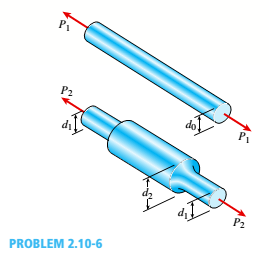# ,10-6 A prismatic bar with a diameter d 0 = 20 mm is being compared with a stepped bar of the same diameter( d | = 20 mm) that is enlarged in the middle region lo a diameter d 2 = 25 mm (see figure). The radius of the fillets in the stepped bar is 2.0 mm. (a) Does enlarging the bar in the middle region make it stronger than the prismatic bar? Demonstrate your answer by determining the maximum permissible load for the prismatic bar and the maximum permissible load F, for the enlarged bar, assuming that the allowable stress for the material is 80 MPa. (b) What should be the diameter d 0 of the prismatic bar if it is to have the same maximum permissible load as does the stepped bar?### Mechanics of Materials (MindTap Co...

9th Edition
Barry J. Goodno + 1 other
Publisher: Cengage Learning
ISBN: 9781337093347
Chapter 2, Problem 2.10.6P
Textbook Problem
66 views

## ,10-6 A prismatic bar with a diameter d0= 20 mm is being compared with a stepped bar of the same diameter(d| = 20 mm) that is enlarged in the middle region lo a diameter d2= 25 mm (see figure). The radius of the fillets in the stepped bar is 2.0 mm.(a) Does enlarging the bar in the middle region make it stronger than the prismatic bar? Demonstrate your answer by determining the maximum permissible load for the prismatic bar and the maximum permissible load F, for the enlarged bar, assuming that the allowable stress for the material is 80 MPa.(b) What should be the diameter d0of the prismatic bar if it is to have the same maximum permissible load as does the stepped bar?i.

To determine

We have to determine that does enlarging the bar in the middle region make it stronger

than the prismatic bar.

### Explanation of Solution

Given information:

d1 = 20mm

d1 = 20mm

d2 = 26mm

Allowable stress, st = 80 Mpa

For prismatic bar, P1= σ1A0= σ1(π4)d02

P1= 80(π4)202= 25.1 N

For stepped bar,

R/d1=0.10, d1/d2= 1.25, K1

ii.

To determine

We have to determine that what should be the diameter d0 of the prismatic bar if it is to have the same maximum permissible load as does the stepped bar.

### Still sussing out bartleby?

Check out a sample textbook solution.

See a sample solution

#### The Solution to Your Study Problems

Bartleby provides explanations to thousands of textbook problems written by our experts, many with advanced degrees!

Get Started

Find more solutions based on key concepts
Name the three ways in which CV joints can be classified.

Automotive Technology: A Systems Approach (MindTap Course List)

What is structural independence, and why is it important?

Database Systems: Design, Implementation, & Management

In what unit is electric current measured?

Engineering Fundamentals: An Introduction to Engineering (MindTap Course List)

What formula can a project manager use to estimate task duration? Provide an example.

Systems Analysis and Design (Shelly Cashman Series) (MindTap Course List)

What is the difference between system and application software?

Enhanced Discovering Computers 2017 (Shelly Cashman Series) (MindTap Course List)

Name two types of respirators and describe how they work.

Welding: Principles and Applications (MindTap Course List)

If your motherboard supports ECC DDR3 memory, can you substitute non-ECC DDR3 memory?

A+ Guide to Hardware (Standalone Book) (MindTap Course List)